Courses

# Facts That Matter- Heron’s Formula Class 9 Notes | EduRev

## Mathematics (Maths) Class 9

Created by: Full Circle

## Class 9 : Facts That Matter- Heron’s Formula Class 9 Notes | EduRev

The document Facts That Matter- Heron’s Formula Class 9 Notes | EduRev is a part of the Class 9 Course Mathematics (Maths) Class 9.
All you need of Class 9 at this link: Class 9

Facts That Matter

• Area of a triangle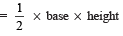• Heron’s formula: For sides of a triangle being a, b, c, the semi-perimeter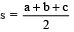and area of the triangle =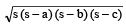•  For finding area of a quadrilateral we divide it into various triangles. Then we use Heron’s formula to find the area of the triangles.

AREA OF TRIANGLES
We have already learnt that area of a triangle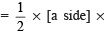[altitude corresponding to that side (or height)]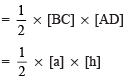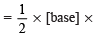[altitude corresponding to base (or height)]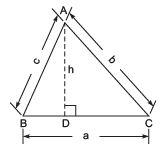Thus, area of a triangle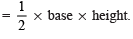Note:
Unit of measurement for area of any plane figure is taken as square metre (m2) or square centimetre (cm2), etc.

HERON’S FORMULA
When it is not possible to find the height of the triangle easily and measures of all the three sides are known then we use Heron’s formula, which is given by
Area of a triangle =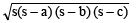where a, b and c are the sides of the triangle and s = semi-perimeter, i.e. half the perimeter of the triangle =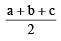AREA OF AN EQUILATERAL TRIANGLE
Let the side of the equilateral triangle be ‘a’.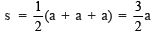⇒ Area of the triangle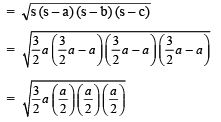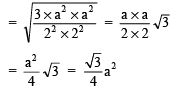Thus, area of an equilateral triangle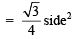## Mathematics (Maths) Class 9

192 videos|230 docs|82 tests

,

,

,

,

,

,

,

,

,

,

,

,

,

,

,

,

,

,

,

,

,

;